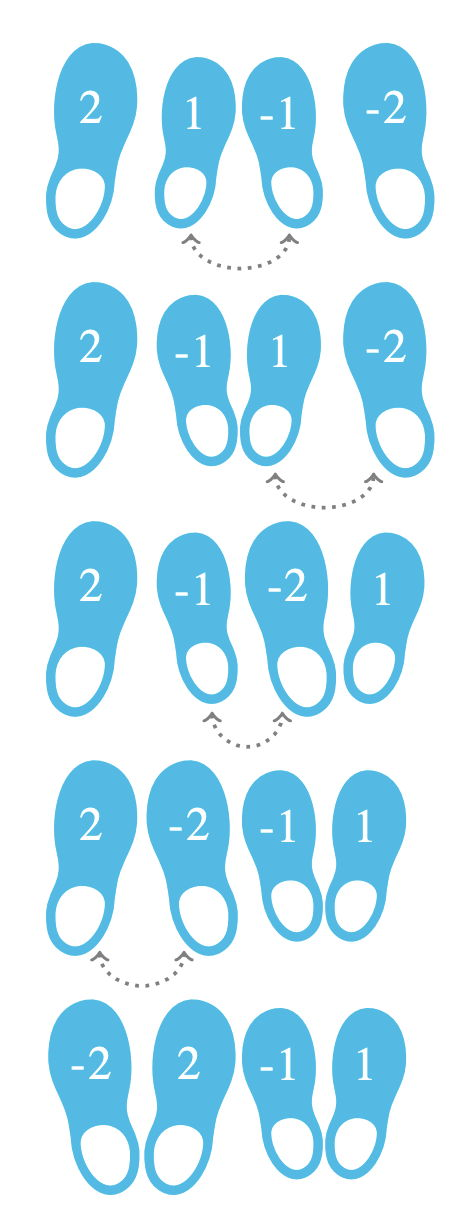시간 제한메모리 제한제출정답맞은 사람정답 비율
1 초 1024 MB68454474.576%

## 문제

Adnan owns the biggest shoe store in Baku. A box containing $n$ pairs of shoes has just arrived at the store. Each pair consists of two shoes of the same size: a left and a right one. Adnan has put all of the $2n$ shoes in a row consisting of $2n$ positions numbered $0$ through $2n-1$ from left to right.

Adnan wants to rearrange the shoes into a valid arrangement. An arrangement is valid if and only if for every $i$ ($0 \leq i \leq n-1$), the following conditions hold:

• The shoes at positions $2i$ and $2i+1$ are of the same size.
• The shoe at position $2i$ is a left shoe.
• The shoe at position $2i+1$ is a right shoe.

For this purpose, Adnan can make a series of swaps.

In each swap, he selects two shoes that are adjacent at that moment and exchanges them (i.e., picks them up and puts each one on the former position of the other shoe).

Two shoes are adjacent if their positions differ by one.

Determine the minimum number of swaps that Adnan needs to perform in order to obtain a valid arrangement of the shoes.

## 구현

You should implement the following procedure:

int64 count_swaps(int[] S)
• $S$: an array of $2n$ integers. For each $i$ ($0 \leq i \leq 2n-1$), $|S[i]|$ is a non-zero value equal to the size of the shoe initially placed at position $i$. Here, $|x|$ denotes the absolute value of $x$, which equals $x$ if $x>0$ and equals $-x$ if $x<0$. If $S[i] < 0$, the shoe at position $i$ is a left shoe; otherwise, it is a right shoe.
• This procedure should return the minimum number of swaps (of adjacent shoes) that need to be performed in order to obtain a valid arrangement.

## 제한

• $1 \leq n \leq 100\,000$
• For each $i$ ($0 \leq i \leq 2n-1$), $1 \leq |S[i]| \leq n$.
• A valid arrangement of the shoes can be obtained by performing some sequence of swaps.

## 예시 1

Consider the following call:

count_swaps([2, 1, -1, -2])

Adnan can obtain a valid arrangement in $4$ swaps.

For instance, he can first swap shoes $1$ and $-1$, then $1$ and $-2$, then $-1$ and $-2$, and finally $2$ and $-2$. He would then obtain the following valid arrangement: $[-2, 2, -1, 1]$. It is not possible to obtain any valid arrangement with less than $4$ swaps. Therefore, the procedure should return $4$.## 예시 2

In the following example, all the shoes have the same size:

count_swaps([-2, 2, 2, -2, -2, 2])

Adnan can swap the shoes at positions $2$ and $3$ to obtain the valid arrangement $[-2, 2, -2, 2, -2, 2]$, so the procedure should return $1$.

## 서브태스크

번호 배점 제한
1 10

$n = 1$

2 20

$n \leq 8$

3 20

All the shoes are of the same size.

4 15

All shoes at positions $0, \ldots, n-1$ are left shoes, and all shoes at positions $n, \ldots, 2n-1$ are right shoes. Also, for each $i$ ($0 \leq i \leq n-1$), the shoes at positions $i$ and $i+n$ are of the same size.

5 20

$n \leq 1000$

6 15

## 출처• 문제를 만든 사람: Danylo Mysak

## 제출할 수 있는 언어

C++17, C++14, C++20, C++14 (Clang), C++17 (Clang), C++20 (Clang)

## 채점 및 기타 정보

• 예제는 채점하지 않는다.